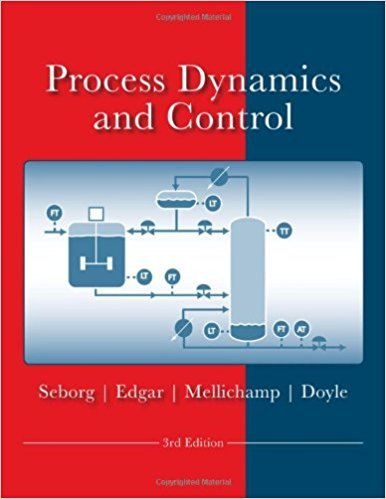×
Get Full Access to Process Dynamics And Control - 3 Edition - Chapter 6 - Problem 6.12
Get Full Access to Process Dynamics And Control - 3 Edition - Chapter 6 - Problem 6.12

×

# For the process described by the exact transfer function 5ISBN: 9780470128671 148

## Solution for problem 6.12 Chapter 6

Process Dynamics and Control | 3rd Edition

• Textbook Solutions
• 2901 Step-by-step solutions solved by professors and subject experts
• Get 24/7 help from StudySoup virtual teaching assistantsProcess Dynamics and Control | 3rd Edition

4 5 1 280 Reviews
13
4
Problem 6.12

For the process described by the exact transfer function 5 G(s) - ------=------- (lOs + 1)(4s + l)(s + 1)(0.2s + 1) (a) Find an approximate transfer function of second-orderplus-time-delay form that describes this process. (b) Simulate and plot the response y(t) of both the approximate model and the exact model on the same graph for a unit step change in input x(t). (c) What is the maximum error between the two responses? Where does it occur?

Step-by-Step Solution:
Step 1 of 3

1 Chapter 12: Composites Materials  Composite materials o Mixture of 2 or more than 2 components o Ex: Concrete, asphalt, wood  Why use composites o Improve properties  Composites made up of 2 components o (1) Matrix  Metals  Metal matrix composite (MMC)  Ceramics  CMC  Polymers  PMC (2) Reinforcement (Reinforcing agent)  Either a particle or a fiber  Stress transfer is the BIGGEST ROLE of reinforcing agent  The applied stress will be dispersed throughout

Step 2 of 3

Step 3 of 3

##### ISBN: 9780470128671

The answer to “For the process described by the exact transfer function 5 G(s) - ------=------- (lOs + 1)(4s + l)(s + 1)(0.2s + 1) (a) Find an approximate transfer function of second-orderplus-time-delay form that describes this process. (b) Simulate and plot the response y(t) of both the approximate model and the exact model on the same graph for a unit step change in input x(t). (c) What is the maximum error between the two responses? Where does it occur?” is broken down into a number of easy to follow steps, and 77 words. The full step-by-step solution to problem: 6.12 from chapter: 6 was answered by , our top Engineering and Tech solution expert on 11/15/17, 04:03PM. This full solution covers the following key subjects: approximate, transfer, process, model, function. This expansive textbook survival guide covers 24 chapters, and 420 solutions. Process Dynamics and Control was written by and is associated to the ISBN: 9780470128671. Since the solution to 6.12 from 6 chapter was answered, more than 286 students have viewed the full step-by-step answer. This textbook survival guide was created for the textbook: Process Dynamics and Control, edition: 3.

Unlock Textbook Solution